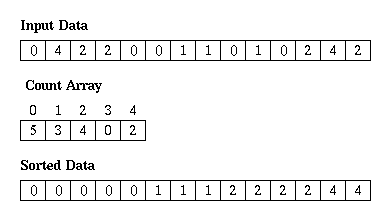# Counting Sort in C

Here you will learn about counting sort in C

Counting sort algorithm is a sorting algorithm which do not involve comparison between elements of an array. In this tutorial I am sharing counting sort program in C. Steps that I am doing to sort the elements are given below.

1. First of all I am reading n elements in array a[]. While reading the array elements I have also calculated the maximum element.

2. Now the actual sorting is done in counting_sort() function. Here I am counting the occurrence of each element in the array a[] and then storing it at the index equal to the value of that element. For example occurrence of element 5 will be stored at index 5, occurrence of element 9 will be stored at index 9 and so on.

3. As the value at each index in count[] array is the occurrence of that index or element, so the elements are printed in ascending order by printing each index number of times equal to its corresponding value.

Also Read: Merge Sort in CCounting Sort Example – Image Source

I have also added a video tutorial below that will help you to understand the counting sort algorithm easily. If you are facing any problem then ask it in the comment section.

## Program for Counting Sort in C

OutputIf you found any mistake or anything missing in above tutorial for counting sort in C then please mention it by commenting below.
Category: Algorithm DSAA crazy computer and programming lover. He spend most of his time in programming, blogging and helping other programming geeks.

## 12 thoughts on “Counting Sort in C”

1.Alexandra

Hei! Thanks for the code! It was very useful for me 🙂 I really appreciate that it is clean and very easy to understand.
though, I found a verry, verry little mistake here and i thought you might want to correct it 😀
printf(“nEnter elements:”); needs a “\”
Thanks again and good luck with what you are doing here 🙂

2.jozko

finally working one

3.chan

Thanks for the post.
However, I wonder this approach gives a ‘Stable sorting’, which is a characteristic of Counting Sort, as it does not actually sort by keys + pointers to records, does it?

4.archu

Give me a simple example for counting problems in c

5.zeeshan ul islam

bro this is very very neat.
used it successfully.

6.kanith

what does the statement count[a[i]]=count[a[i]]+1; mean?

7.Steffi Bunny

Your tutorials are very lucid and interesting. Keep it up!

8.Rizavia

the program is not working when all the numbers are two or more digits…. 🙁

1.Hasan Shahriar Boni

good program. but it is not working for the number 50 and above. this can be solved by increasing the value of the count array..

9.Abhishek Rana

This is not counting sort. Because it does not have complexity (n).

10.shoutnine

keep it up ! really helped me 🙂

11.Shubham Jain

Where are you modifying or copying the value to original array?